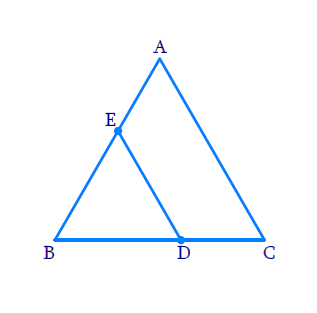# Ex.6.4 Q8 Triangles Solution - NCERT Maths Class 10

Go back to  'Ex.6.4'

## Question

$$ABC$$ and $$BDE$$ are two equilateral triangles such that $$D$$ is the mid-point of $$BC.$$ Ratio of the areas of triangles $$ABC$$ and $$BDE$$ is

(a) $$2 : 1$$

(b) $$1 : 2$$

(c) $$4 : 1$$

(d) $$1 : 4$$Video Solution
Triangles
Ex 6.4 | Question 8

## Text Solution

Reasoning:

$$AAA$$ criterion.

Steps:

$$\Delta A B C \sim \Delta B D E$$ $$(\because \text{equilateral triangles)}$$

The ratio of the areas of two similar triangles is equal to the square of the ratio of their corresponding sides.

\begin{aligned} \frac{ar\Delta A B C}{ar \Delta B D E} &=\frac{(B C)^{2}}{(B D)^{2}} \\ &=\frac{(B C)^{2}}{\left(\frac{B C}{2}\right)^{2}} \\ [ D \text { is the} & \text{ mid point} \text{ of } BC] \\ &=\frac{(B C)^{2} \times 4}{(B C)^{2}}\\&=4 \end{aligned}

\begin{aligned} ar\Delta ABE : ar\Delta BDE =4:1\end{aligned}

The answer is (c) $$4:1$$

Learn from the best math teachers and top your exams

• Live one on one classroom and doubt clearing
• Practice worksheets in and after class for conceptual clarity
• Personalized curriculum to keep up with school